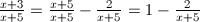# Combined Fuel Economy, Day 2 of 2

2 teachers like this lesson
Print Lesson

## Objective

SWBAT use rational functions to model fuel economy. SWBAT rewrite rational expressions in different forms and interpret the differences.

#### Big Idea

Miles per gallon? Sounds like an opportunity for modeling with rational functions!

## Setting the Stage

5 minutes

Quickly: Do you recall the fuel efficiency problem we were working on yesterday?

Students should be able to quickly lay out the basic scenario and describe the situation they are investigating.  Namely, they're looking into the combined fuel economy of a typical car whose highway fuel economy is 10 mpg greater than its city fuel economy.  To do this, we've taken the standard formula and subsititued (x + 10) for y.

Before turning students loose, I'll emphasize that when students are asked to "show" something in the final problem, they should try to show it in at least three ways: numerically, graphically, and analytically.  That is, I'd like to see them making a data table, making a graph, and drawing conclusions from the structure of the equation. (See this video for my thoughts on how this exemplifies MP 1.)

## Solving Problems

30 minutes

Picking up where they left off in the problem set, Combined Fuel Economy, students should work individually or in small groups.  Today's primary tasks are to find some means of transforming the equation from one form into another (question 3) and then to use that new form to draw conclusions about the end behavior (question 4).

I will encourage students to use synthetic division or the method of completing the square if they are stuck on question 3.  I might also use another example, such asto help get them started.

As students begin to draw conclusions about the end behavior of this function, I will remind them to "show" the end behavior with a data table (once x grows beyond 20, the conclusion becomes reasonable), a graph (look for an oblique asymptote), and by examining the structure of the equation.  This last point is the most difficult; students must recognize that as the denominator grows, the final term approaches zero. (MP 1)

This problem set is adapted from Illustrative_Mathematics; please refer to this for the solutions and some excellent commentary and solutions.

## Wrapping Up

10 minutes

Hopefully, by the end of this lesson, the bulk of the class will have reached their final conclusions.  They should have produced fine looking graphs, small-but-significant tables of data, and a decent analysis of the structure of the equation.

I would like one group to share their solution with the class via document camera.  We will begin with the data table to see that the combined fuel economy approaches (x + 5).  We will move next to the equation itself to show that its structure leads to the same conclusion.  Finally, we will examine the graph to see that the oblique asymptote is the line y = x + 5.  During this conversation, I'll make sure that students are constantly interpreting their mathematical statements and conclusions in terms of the fuel efficiency situation. (MP 4)

A 3-2-1 Exit Ticket is a great formative assessment as students head out the door.